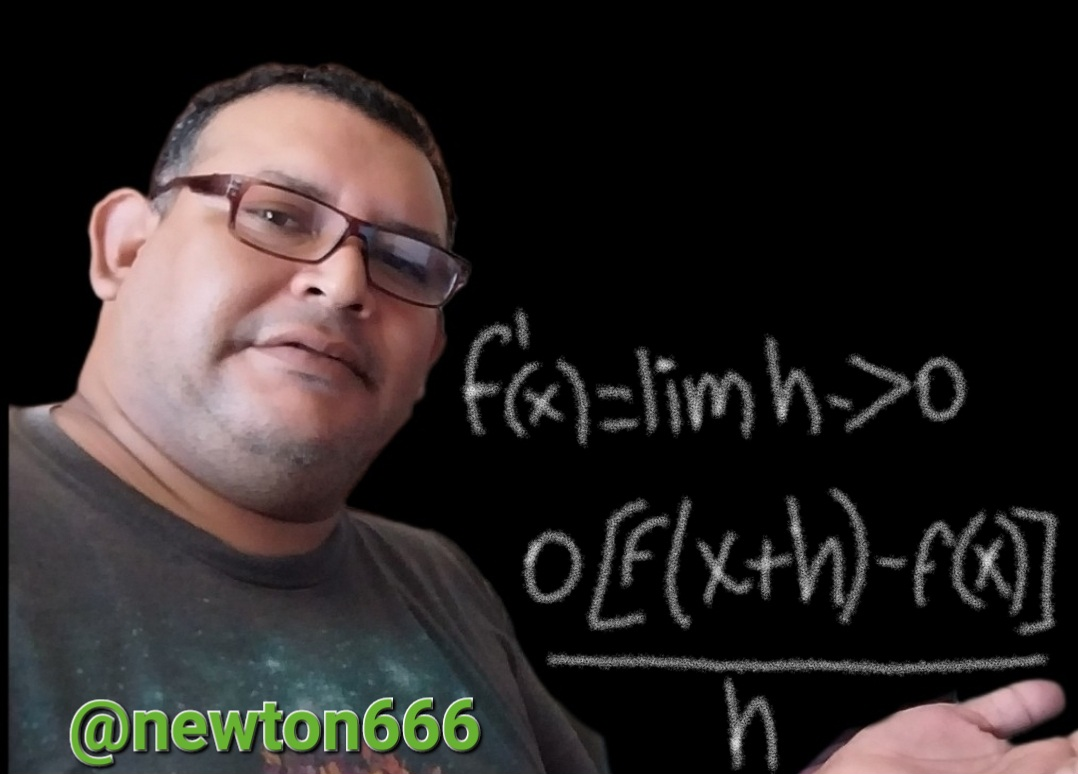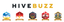# Application of derivatives in the field of physics

in StemSocial2 months ago
Greetings, science lover friends, it is beneficial to be aware of the value of mathematical and calculation tools in addressing a variety of physical phenomena. Today, I'll provide two particularly significant and original instances for their use and explanation. The derivative is a fundamental mathematical technique that is utilized in many areas of physics, my dear reader buddies.The derivative in physics is a term used to indicate how quickly one variable changes as a function of another. For instance, flow rate is the derivative of volume with respect to time and is used to calculate the slope of a curve, which is useful in physics to analyze the shape of a function, the rate of change of a magnitude, etc. Velocity is the derivative of position with respect to time, acceleration is the derivative of velocity with respect to time, and so on.

The mathematical definition of a function's derivative is referred to as the "mother equation" of the derivative. The instantaneous rate of change of a function at a specific location is known as the derivative of a function f(x).
The mother equation of the derivative is written as follows:

f'(x) = lim h->0 [f(x+h) - f(x)] / h

Where h denotes an incredibly small value and f'(x) denotes the function's derivative at the point x.

In other words, according to the parent derivative equation, the derivative of a function at a specific point can be determined by figuring out how quickly the function is changing as the change in x gets closer to zero. Since it enables us to compute the instantaneous rates of change of functions at certain places and comprehend the behavior of functions at various sites, this equation is crucial for differential calculus.

What we have is as follows: a straight-moving object whose position in time is determined by the function:

x(t) = 3t^2 - 2t + 1

Where t is the time in seconds and x is the position in meters.

The pace at which the object's position changes in relation to time is known as its velocity. Hence, we must calculate the position function with respect to time in order to determine the velocity at any given instant in time:

v(t) = dx/dt = d/dt(3t^2 - 2t + 1)

To derive the function, we apply the power rule and the addition rule:

v(t) = 6t - 2

Thus, the function gives the object's speed at any given instant of time:

v(t) = 6t - 2

The derivative gives us a way to express velocity, which in this case is the rate at which an object's location changes as a function of time. The object's speed varies at a fixed rate of 6 m/s per second.

Any electrically charged object is surrounded by the electric field, a real physical field that stretches throughout space. The force that a test charge would encounter if it were placed anyplace in space is used to characterize this field. The volt per meter (V/m), which serves as the unit of measurement, is a vector that depicts the direction and strength of the electric field at a given position.

The magnetic field, on the other hand, is a physical field that also reaches into space and is created by electrical charges, such as electrons, that are moving. The force that a moving charge would encounter if it were placed at any location in space is used to explain this area. A vector that depicts the strength and direction of the magnetic field at a certain location uses the tesla as its unit of measurement (T).

Both fields are a part of electromagnetism and are intimately related to one another. Maxwell's equations, which indicate that a change in the electric field generates a magnetic field and a change in the magnetic field generates an electric field, describe the interaction between the electric field and the magnetic field. Several gadgets and technologies, like electric motors and transformers, operate as a result of this interaction.

When describing the behavior of physical fields, the derivative is a highly helpful mathematical tool. For instance, we can use it to estimate the variance of the electric or magnetic field at a specific location or to figure out how quickly these fields are changing over time. I'll then give an illustration of how to use the derivative to determine how the electric field changes at a specific location.

Assume that we wish to determine the variation in the electric field at a point P situated at a distance r from the charge. We have a point charge q at the origin of the coordinate system. We are aware of the electric field produced by a point charge at a point P is given by the expression:

E = k * q / r^2

Where r is the distance between the charge and point P, q is the charge on the particle, and k is Coulomb's constant.

We can use the derivative to determine how the electric field changes at a given point P. The derivative of E with regard to r in this instance is provided by:

dE/dr = -2kq*/r3

According to this expression, the rate of change of the electric field is inversely proportional to the cube of the distance as a function of the distance r. In other words, the electric field at a given spot will vary more widely the closer a charged object is to it.

The derivative is a highly helpful mathematical tool for describing how physical fields like the magnetic and electric fields behave. By applying the derivative, we can compute the rate of change of these fields as a function of time or distance, allowing us to better understand their behavior and apply this information in practice.

Photo edited by my Samsung A23 phone

Bibliography Reference

Physics for Science and Engineering by Raymond A. Serway and John W. Jewett Jr, 2010.

University Physics by Francis Sears and Mark Zemansky 2002.

Classical Mechanics by Herbert Goldstein 1980.

Electricity and Magnetism" by Edward M. Purcell and David J. Morin 2013.Sort:

Congratulations @newton666! You have completed the following achievement on the Hive blockchain And have been rewarded with New badge(s)You distributed more than 29000 upvotes.Your next target is to reach 30000 upvotes.

If you no longer want to receive notifications, reply to this comment with the word `STOP`The Hive Gamification Proposal